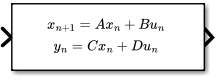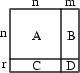# Discrete State-Space

Implement discrete state-space system

• Library:## Description

### Block Behavior for Non-Empty Matrices

The Discrete State-Space block implements the system described by

`$\begin{array}{c}x\left(n+1\right)=Ax\left(n\right)+Bu\left(n\right)\\ y\left(n\right)=Cx\left(n\right)+Du\left(n\right),\end{array}$`

where u is the input, x is the state, and y is the output. The matrix coefficients must have these characteristics, as illustrated in the following diagram:

• A must be an n-by-n matrix, where n is the number of states.

• B must be an n-by-m matrix, where m is the number of inputs.

• C must be an r-by-n matrix, where r is the number of outputs.

• D must be an r-by-m matrix.The block accepts one input and generates one output. The width of the input vector is the number of columns in the B and D matrices. The width of the output vector is the number of rows in the C and D matrices. To define the initial state vector, use the Initial conditions parameter.

To specify a vector or matrix of zeros for A, B, C, D, or Initial conditions, use the `zeros` function.

### Block Behavior for Empty Matrices

When the matrices A, B, and C are empty (for example, `[]`), the functionality of the block becomes `y(n) = Du(n)`. If the Initial conditions vector is also empty, the block uses an initial state vector of zeros.

## Ports

### Input

expand all

Input vector, where the width equals the number of columns in the B and D matrices. For more information, see Description.

### Tip

For integer and fixed-point input signals, use the Fixed-Point State-Space block.

Data Types: `single` | `double`

### Output

expand all

Output vector, with width equal to the number of rows in the C and D matrices. For more information, see Description.

Data Types: `single` | `double`

## Parameters

expand all

### Main

Specify the matrix coefficient `A`, as a real-valued n-by-n matrix, where n is the number of states. For more information on the matrix coefficients, see Description.

#### Programmatic Use

 Block Parameter: `A` Type: character vector Values: scalar | vector | matrix Default: `'1'`

Specify the matrix coefficient `B`, as a real-valued n-by-m matrix, where n is the number of states, and m is the number of inputs. For more information on the matrix coefficients, see Description.

#### Programmatic Use

 Block Parameter: `B` Type: character vector Values: scalar | vector | matrix Default: `'1'`

Specify the matrix coefficient C, as a real-valued r-by-n matrix, where r is the number of outputs, and n is the number of states. For more information on the matrix coefficients, see Description.

#### Programmatic Use

 Block Parameter: `C` Type: character vector Values: scalar | vector | matrix Default: `'1'`

Specify the matrix coefficient D, as a real-valued r-by-m matrix, where r is the number of outputs, and m is the number of inputs. For more information on the matrix coefficients, see Description.

#### Programmatic Use

 Block Parameter: `D` Type: character vector Values: scalar | vector | matrix Default: `'1'`

Specify the initial state vector as a scalar or vector. The initial state vector cannot include `inf` or `NaN` values.

#### Programmatic Use

 Block Parameter: `InitialCondition` Type: character vector Values: scalar | vector Default: `'0'`

Specify the time interval between samples. See Specify Sample Time.

#### Programmatic Use

 Block Parameter: `SampleTime` Type: character vector Values: scalar | vector Default: `'-1'`

### State Attributes

Use this parameter to assign a unique name to the block state. The default is `' '`. When this field is blank, no name is assigned. When using this parameter, remember these considerations:

• A valid identifier starts with an alphabetic or underscore character, followed by alphanumeric or underscore characters.

• The state name applies only to the selected block.

This parameter enables State name must resolve to Simulink signal object when you click Apply.

For more information, see Apply Storage Classes to Individual Signal, State, and Parameter Data Elements (Simulink Coder).

#### Programmatic Use

 Block Parameter: `StateName` Type: character vector Values: unique name Default: `''`

Select this check box to require that the state name resolves to a Simulink® signal object.

#### Dependencies

To enable this parameter, specify a value for State name. This parameter appears only if you set the model configuration parameter Signal resolution to a value other than `None`.

Selecting this check box disables Code generation storage class.

#### Programmatic Use

 Block Parameter: `StateMustResolveToSignalObject` Type: character vector Values: `'off' | 'on'` Default: `'off'`

Choose a custom storage class package by selecting a signal object class that the target package defines. For example, to apply custom storage classes from the built-in package `mpt`, select `mpt.Signal`. Unless you use an ERT-based code generation target with Embedded Coder®, custom storage classes do not affect the generated code.

If the class that you want does not appear in the list, select `Customize class lists`. For instructions, see Target Class Does Not Appear in List of Signal Object Classes (Embedded Coder).

For information about storage classes, see Apply Storage Classes to Individual Signal, State, and Parameter Data Elements (Simulink Coder). For information about custom storage classes, see Apply Built-In and Customized Storage Classes to Data Elements (Embedded Coder).

#### Programmatic Use

 Block Parameter: `StateSignalObject` Type: character vector Values: ```'Simulink.Signal' | ''``` Default: `'Simulink.Signal'`

Select state storage class for code generation.

Use Signal object class to select custom storage classes from a package other than `Simulink`.

#### Dependencies

To enable this parameter, specify a value for State name.

#### Programmatic Use

 Block Parameter: `StateStorageClass` Type: character vector Values: ```'Auto' | 'Model default' | 'ExportedGlobal' | 'ImportedExtern' | 'ImportedExternPointer' | 'Custom' | ...``` Default: `'Auto'`

Specify a storage type qualifier such as `const` or `volatile`.

### Note

TypeQualifier will be removed in a future release. To apply storage type qualifiers to data, use custom storage classes and memory sections. Unless you use an ERT-based code generation target with Embedded Coder, custom storage classes and memory sections do not affect the generated code.

During simulation, the block uses the following values:

• The initial value of the signal object to which the state name is resolved

• Minimum and Maximum values of the signal object

#### Dependencies

To enable this parameter, set Code generation storage class to `ExportedGlobal`, `ImportedExtern`, `ImportedExternPointer`, or `Model default`. This parameter is hidden unless you previously set its value.

#### Programmatic Use

 Block Parameter: `RTWStateStorageTypeQualifier` Type: character vector Values: ```'' | 'const' | 'volatile' | ...``` Default: `''`

## Block Characteristics

 Data Types `double` | `single` Direct Feedthrough `yes` Multidimensional Signals `no` Variable-Size Signals `no` Zero-Crossing Detection `no`